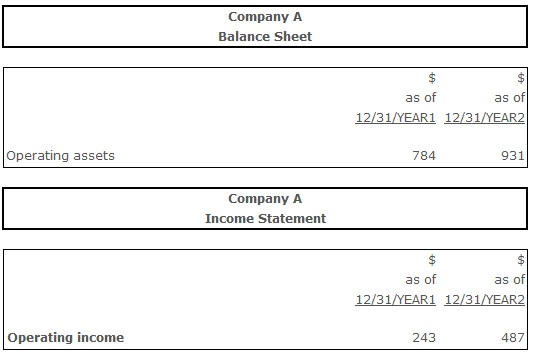# Return on Operating Assets

Return on Operating Assets – a ratio indicating how efficiently company utilizes its revenue generating kind of assets (assets, used in the ongoing operations of a company). Commonly being computed by the company's management to measure the operating earnings, generated by the revenue generating assets, and to consider minimizing usage of all other kinds of assets (the ones that do not contribute to the revenue). The computation includes such assets as cash, accounts receivable, prepaid expenses, inventories etc. In other words, return on operating assets ratio is a measure of firm's operating profitability. Obviously, company would be interested in higher values of this ratio, as this would indicate the efficient use of the revenue generating assets.

## Formula(s):

Return on Operating Assets = Operating Income ÷ Average Operating Assets

Return on Operating Assets = Operating Income ÷ (Total Assets - Construction in Progress – Identifiable Intangible Assets - Net - Goodwill - Deferred Income Taxes and Other Assets)

## Example:Return on Operating Assets (Year 1) = 243 ÷ 784 = 0,30 = 30%

Return on Operating Assets (Year 2) = 487 ÷ 931 = 0,52 = 52%

According to the calculations, the operating assets were yielding a 30% return in year 1. In year 2 it increased to 50%, indicating positive trend in terms of company’s profitability and operating assets utilization efficiency.

## Conclusion:

To measure company's operating profitability and operating assets utilization efficiency the return on operating assets is being computed. Higher ratios indicate higher profitability, while ratios below 1 mean unefficient use of the operating assets.